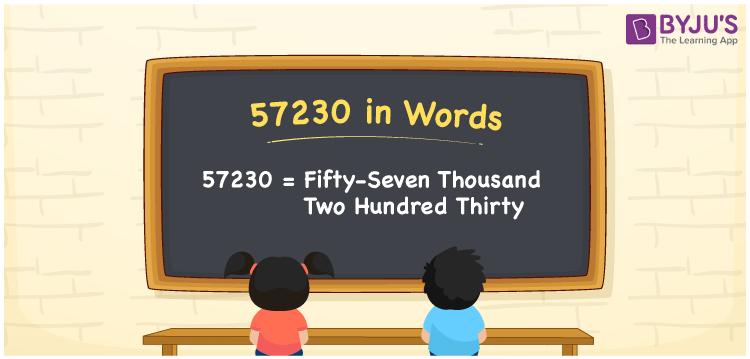# 57230 in Words

57230 in words is written as Fifty-seven thousand two hundred thirty In both the International System of Numerals and the Indian System of Numerals, 57230 is written as Fifty-seven thousand two hundred thirty The number 57230 is a Cardinal Number as it denotes some quantity. For example, “57230 candidates are going to apply for the position ”.

 57230 in Words Fifty-seven thousand two hundred thirty Fifty-seven thousand two hundred thirty in Number 57230

## 57230 in English Words

57230 in English words is read as “Fifty-seven thousand two hundred thirty”.## How to Write 57230 in Words?

To write 57230 in words, we shall use the place value chart. In the place value chart, put 5 in the ten thousands, 7 in the thousands, 2 in the hundreds, 3 in the tens and 0 in the ones. Let us make a place value chart to write the number 57230 in words.

 Ten Thousands Thousands Hundreds Tens Ones 5 7 2 3 0

Thus, we can write the expanded form as

5 × Ten Thousand + 7 × Thousand + 2 × Hundred + 3 × Ten + 0 × One

= 5 × 10000 + 7 × 1000 + 2 × 100 + 3 × 10 + 0 × 1

= 50000 + 7000 + 200 + 30 + 0

= 57230

= Fifty-seven thousand two hundred thirty

57230 is a natural number, the successor of 57229 and the predecessor of 57231.

57230 in words – Fifty-seven thousand two hundred thirty

• Is 57230 an odd number? – No
• Is 57230 an even number? – Yes
• Is 57230 a perfect square number? – No
• Is 57230 a perfect cube number? – No
• Is 57230 a prime number? – No
• Is 57230 a composite number? – Yes

## Frequently Asked Questions on 57230 in Words

Q1

### How to write 57230 in words?

57230 in words is written as Fifty-seven thousand two hundred thirty.
Q2

### How to write 57230 in the International and Indian System of Numerals?

In both, the system of numerals, 57230 in words, is written as Fifty-seven thousand two hundred thirty.
Q3

### How to write 57230 in a place value chart?

In the place value chart, write 5 in the ten thousands, 7 in the thousands, 2 in the hundreds, 3 in the tens and 0 in the ones, respectively.